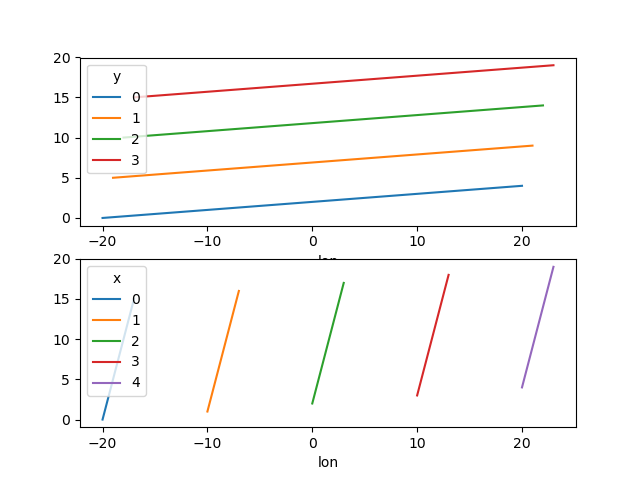# Plotting¶

## Introduction¶

Labeled data enables expressive computations. These same labels can also be used to easily create informative plots.

xarray’s plotting capabilities are centered around `xarray.DataArray` objects. To plot `xarray.Dataset` objects simply access the relevant DataArrays, ie `dset['var1']`. Here we focus mostly on arrays 2d or larger. If your data fits nicely into a pandas DataFrame then you’re better off using one of the more developed tools there.

xarray plotting functionality is a thin wrapper around the popular matplotlib library. Matplotlib syntax and function names were copied as much as possible, which makes for an easy transition between the two. Matplotlib must be installed before xarray can plot.

To use xarray’s plotting capabilities with time coordinates containing `cftime.datetime` objects nc-time-axis v1.2.0 or later needs to be installed.

For more extensive plotting applications consider the following projects:

• Seaborn: “provides a high-level interface for drawing attractive statistical graphics.” Integrates well with pandas.

• HoloViews and GeoViews: “Composable, declarative data structures for building even complex visualizations easily.” Includes native support for xarray objects.

• hvplot: `hvplot` makes it very easy to produce dynamic plots (backed by `Holoviews` or `Geoviews`) by adding a `hvplot` accessor to DataArrays.

• Cartopy: Provides cartographic tools.

### Imports¶

The following imports are necessary for all of the examples.

```In : import numpy as np

In : import pandas as pd

In : import matplotlib.pyplot as plt

In : import xarray as xr
```

For these examples we’ll use the North American air temperature dataset.

```In : airtemps = xr.tutorial.open_dataset('air_temperature')

In : airtemps
Out:
<xarray.Dataset>
Dimensions:  (lat: 25, lon: 53, time: 2920)
Coordinates:
* lat      (lat) float32 75.0 72.5 70.0 67.5 65.0 ... 25.0 22.5 20.0 17.5 15.0
* lon      (lon) float32 200.0 202.5 205.0 207.5 ... 322.5 325.0 327.5 330.0
* time     (time) datetime64[ns] 2013-01-01 ... 2014-12-31T18:00:00
Data variables:
air      (time, lat, lon) float32 ...
Attributes:
Conventions:  COARDS
title:        4x daily NMC reanalysis (1948)
description:  Data is from NMC initialized reanalysis\n(4x/day).  These a...
platform:     Model
references:   http://www.esrl.noaa.gov/psd/data/gridded/data.ncep.reanaly...

# Convert to celsius
In : air = airtemps.air - 273.15

# copy attributes to get nice figure labels and change Kelvin to Celsius
In : air.attrs = airtemps.air.attrs

In : air.attrs['units'] = 'deg C'
```

Note

Until GH1614 is solved, you might need to copy over the metadata in `attrs` to get informative figure labels (as was done above).

## One Dimension¶

### Simple Example¶

The simplest way to make a plot is to call the `xarray.DataArray.plot()` method.

```In : air1d = air.isel(lat=10, lon=10)

In : air1d.plot()
Out: [<matplotlib.lines.Line2D at 0x7f5b93a527f0>]
```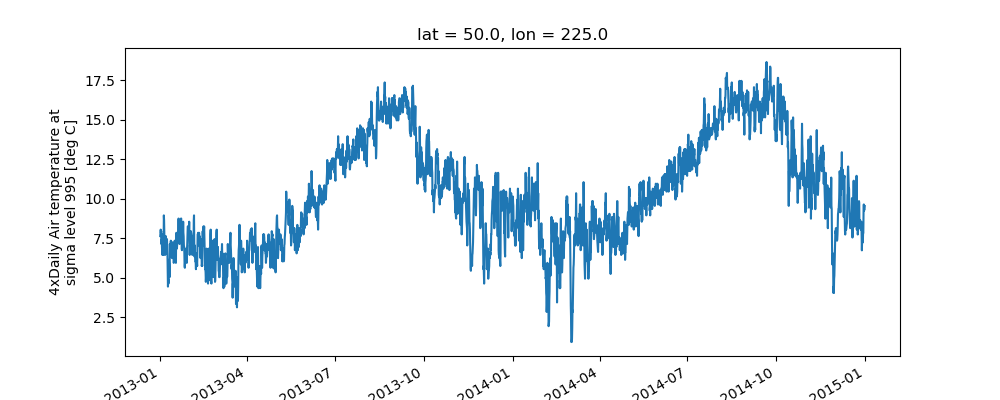xarray uses the coordinate name along with metadata `attrs.long_name`, `attrs.standard_name`, `DataArray.name` and `attrs.units` (if available) to label the axes. The names `long_name`, `standard_name` and `units` are copied from the CF-conventions spec. When choosing names, the order of precedence is `long_name`, `standard_name` and finally `DataArray.name`. The y-axis label in the above plot was constructed from the `long_name` and `units` attributes of `air1d`.

```In : air1d.attrs
Out:
OrderedDict([('long_name', '4xDaily Air temperature at sigma level 995'),
('units', 'deg C'),
('precision', 2),
('GRIB_id', 11),
('GRIB_name', 'TMP'),
('var_desc', 'Air temperature'),
('dataset', 'NMC Reanalysis'),
('level_desc', 'Surface'),
('statistic', 'Individual Obs'),
('parent_stat', 'Other'),
('actual_range', array([185.16, 322.1 ], dtype=float32))])
```

Additional arguments are passed directly to the matplotlib function which does the work. For example, `xarray.plot.line()` calls matplotlib.pyplot.plot passing in the index and the array values as x and y, respectively. So to make a line plot with blue triangles a matplotlib format string can be used:

```In : air1d[:200].plot.line('b-^')
Out: [<matplotlib.lines.Line2D at 0x7f5b93a819e8>]
```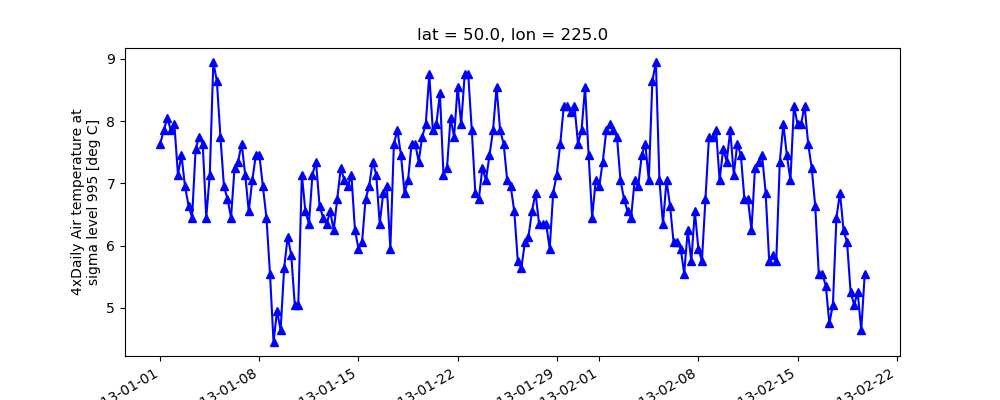Note

Not all xarray plotting methods support passing positional arguments to the wrapped matplotlib functions, but they do all support keyword arguments.

Keyword arguments work the same way, and are more explicit.

```In : air1d[:200].plot.line(color='purple', marker='o')
Out: [<matplotlib.lines.Line2D at 0x7f5b93a49898>]
```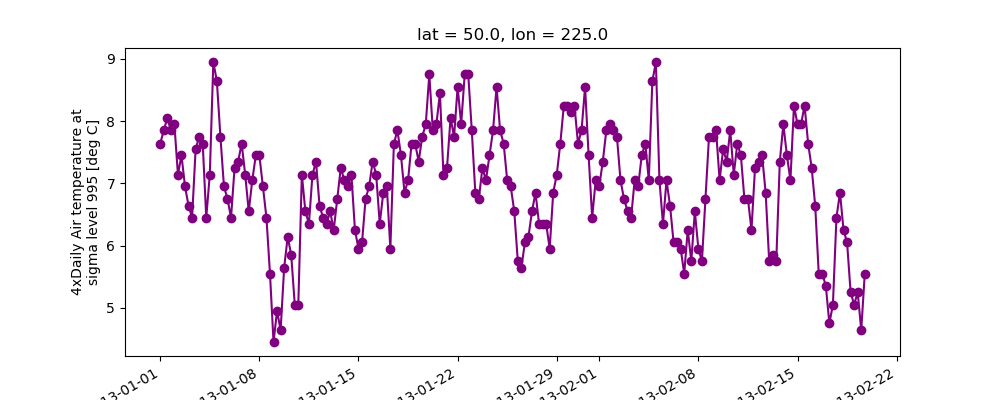To add the plot to an existing axis pass in the axis as a keyword argument `ax`. This works for all xarray plotting methods. In this example `axes` is an array consisting of the left and right axes created by `plt.subplots`.

```In : fig, axes = plt.subplots(ncols=2)

In : axes
Out:
array([<matplotlib.axes._subplots.AxesSubplot object at 0x7f5b93a4d208>,
<matplotlib.axes._subplots.AxesSubplot object at 0x7f5b93996d68>], dtype=object)

In : air1d.plot(ax=axes)
Out: [<matplotlib.lines.Line2D at 0x7f5b939ebcc0>]

In : air1d.plot.hist(ax=axes)
Out:
(array([  9.,  38., 255., 584., 542., 489., 368., 258., 327.,  50.]),
array([ 0.95 ,  2.719,  4.488, ..., 15.102, 16.871, 18.64 ], dtype=float32),
<a list of 10 Patch objects>)

In : plt.tight_layout()

In : plt.draw()
```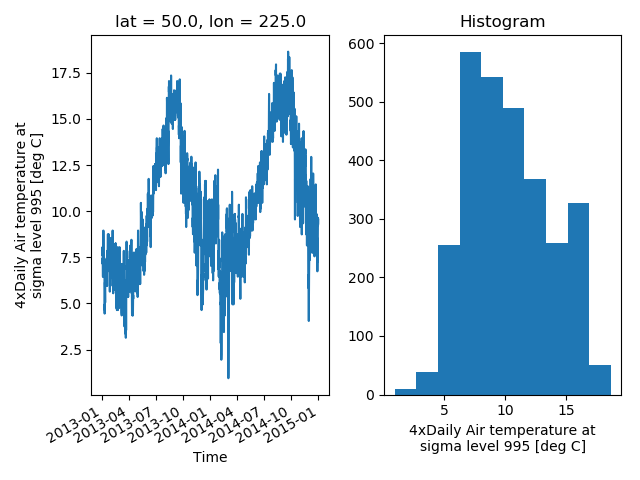On the right is a histogram created by `xarray.plot.hist()`.

### Controlling the figure size¶

You can pass a `figsize` argument to all xarray’s plotting methods to control the figure size. For convenience, xarray’s plotting methods also support the `aspect` and `size` arguments which control the size of the resulting image via the formula `figsize = (aspect * size, size)`:

```In : air1d.plot(aspect=2, size=3)
Out: [<matplotlib.lines.Line2D at 0x7f5b93996898>]

In : plt.tight_layout()
```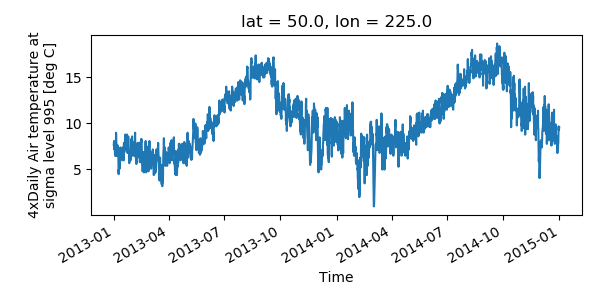This feature also works with Faceting. For facet plots, `size` and `aspect` refer to a single panel (so that `aspect * size` gives the width of each facet in inches), while `figsize` refers to the entire figure (as for matplotlib’s `figsize` argument).

Note

If `figsize` or `size` are used, a new figure is created, so this is mutually exclusive with the `ax` argument.

Note

The convention used by xarray (`figsize = (aspect * size, size)`) is borrowed from seaborn: it is therefore not equivalent to matplotlib’s.

### Multiple lines showing variation along a dimension¶

It is possible to make line plots of two-dimensional data by calling `xarray.plot.line()` with appropriate arguments. Consider the 3D variable `air` defined above. We can use line plots to check the variation of air temperature at three different latitudes along a longitude line:

```In : air.isel(lon=10, lat=[19,21,22]).plot.line(x='time')
Out:
[<matplotlib.lines.Line2D at 0x7f5b93928550>,
<matplotlib.lines.Line2D at 0x7f5b9393d908>,
<matplotlib.lines.Line2D at 0x7f5b9393da90>]
```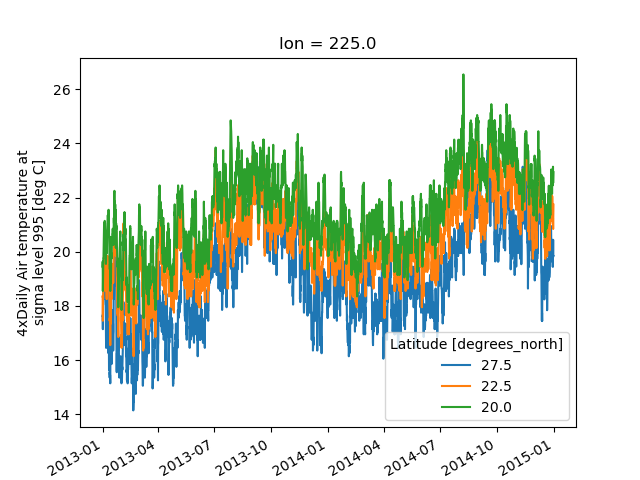It is required to explicitly specify either

1. `x`: the dimension to be used for the x-axis, or

2. `hue`: the dimension you want to represent by multiple lines.

Thus, we could have made the previous plot by specifying `hue='lat'` instead of `x='time'`. If required, the automatic legend can be turned off using `add_legend=False`. Alternatively, `hue` can be passed directly to `xarray.plot()` as air.isel(lon=10, lat=[19,21,22]).plot(hue=’lat’).

### Dimension along y-axis¶

It is also possible to make line plots such that the data are on the x-axis and a dimension is on the y-axis. This can be done by specifying the appropriate `y` keyword argument.

```In : air.isel(time=10, lon=[10, 11]).plot(y='lat', hue='lon')
Out:
[<matplotlib.lines.Line2D at 0x7f5b938f7668>,
<matplotlib.lines.Line2D at 0x7f5b938f76a0>]
```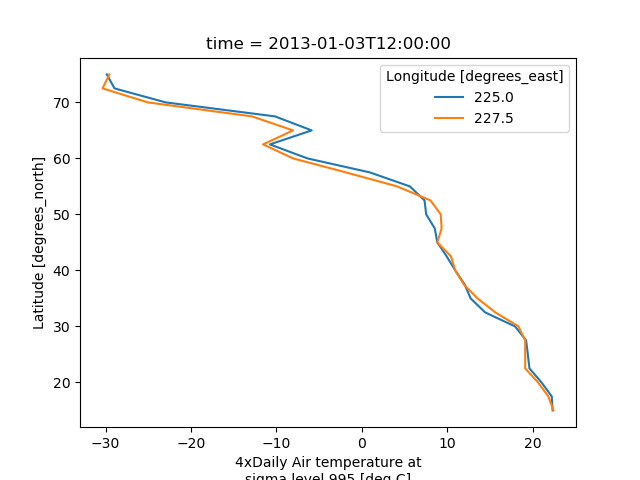### Step plots¶

As an alternative, also a step plot similar to matplotlib’s `plt.step` can be made using 1D data.

```In : air1d[:20].plot.step(where='mid')
Out: [<matplotlib.lines.Line2D at 0x7f5b938f7278>]
```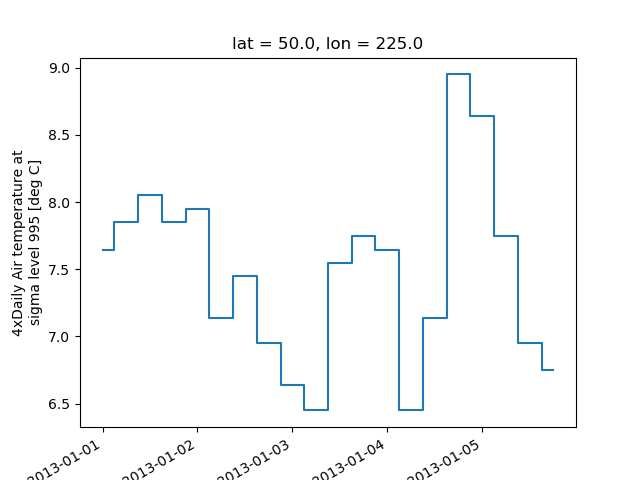The argument `where` defines where the steps should be placed, options are `'pre'` (default), `'post'`, and `'mid'`. This is particularly handy when plotting data grouped with `xarray.Dataset.groupby_bins()`.

```In : air_grp = air.mean(['time','lon']).groupby_bins('lat',[0,23.5,66.5,90])

In : air_mean = air_grp.mean()

In : air_std = air_grp.std()

In : air_mean.plot.step()
Out: [<matplotlib.lines.Line2D at 0x7f5bdd0e72e8>]

In : (air_mean + air_std).plot.step(ls=':')
Out: [<matplotlib.lines.Line2D at 0x7f5b9390eb38>]

In : (air_mean - air_std).plot.step(ls=':')
Out: [<matplotlib.lines.Line2D at 0x7f5b9390ed68>]

In : plt.ylim(-20,30)
Out: (-20, 30)

In : plt.title('Zonal mean temperature')
Out: Text(0.5, 1.0, 'Zonal mean temperature')
```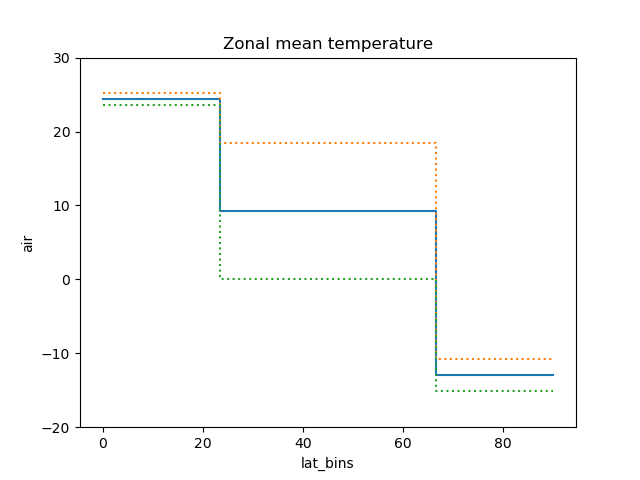In this case, the actual boundaries of the bins are used and the `where` argument is ignored.

## Other axes kwargs¶

The keyword arguments `xincrease` and `yincrease` let you control the axes direction.

```In : air.isel(time=10, lon=[10, 11]).plot.line(y='lat', hue='lon', xincrease=False, yincrease=False)
Out:
[<matplotlib.lines.Line2D at 0x7f5bdcf88518>,
<matplotlib.lines.Line2D at 0x7f5bdcf88fd0>]
```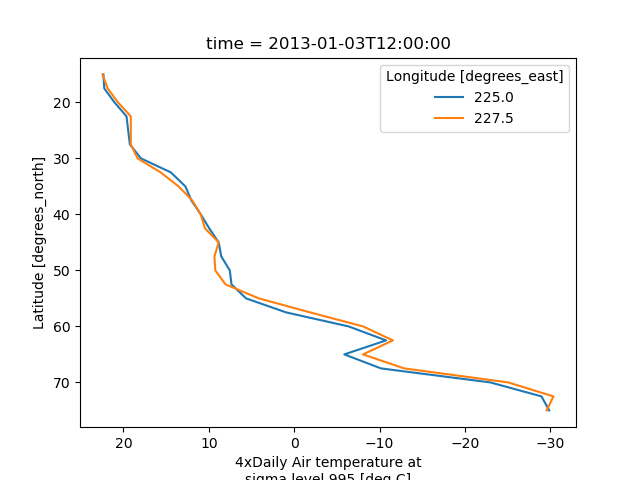In addition, one can use `xscale, yscale` to set axes scaling; `xticks, yticks` to set axes ticks and `xlim, ylim` to set axes limits. These accept the same values as the matplotlib methods `Axes.set_(x,y)scale()`, `Axes.set_(x,y)ticks()`, `Axes.set_(x,y)lim()` respectively.

## Two Dimensions¶

### Simple Example¶

The default method `xarray.DataArray.plot()` calls `xarray.plot.pcolormesh()` by default when the data is two-dimensional.

```In : air2d = air.isel(time=500)

In : air2d.plot()
```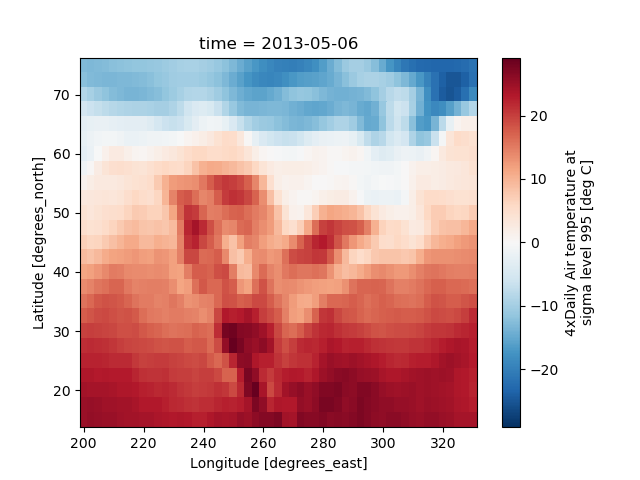All 2d plots in xarray allow the use of the keyword arguments `yincrease` and `xincrease`.

```In : air2d.plot(yincrease=False)
```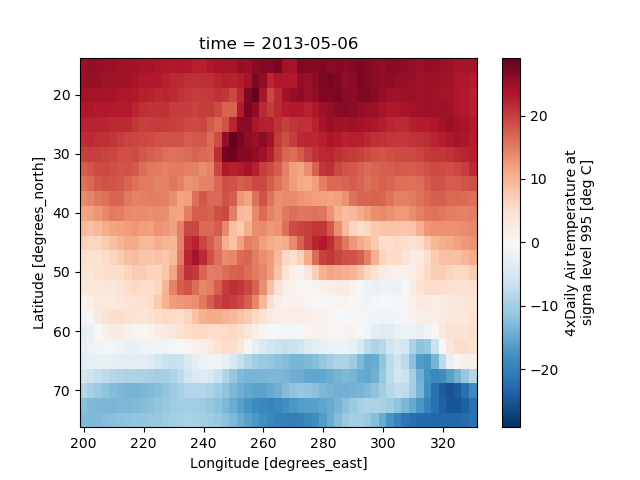Note

We use `xarray.plot.pcolormesh()` as the default two-dimensional plot method because it is more flexible than `xarray.plot.imshow()`. However, for large arrays, `imshow` can be much faster than `pcolormesh`. If speed is important to you and you are plotting a regular mesh, consider using `imshow`.

### Missing Values¶

xarray plots data with Missing values.

```In : bad_air2d = air2d.copy()

In : bad_air2d[dict(lat=slice(0, 10), lon=slice(0, 25))] = np.nan

```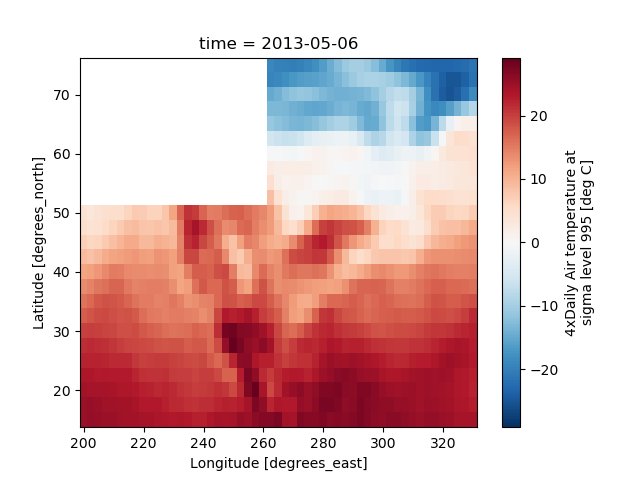### Nonuniform Coordinates¶

It’s not necessary for the coordinates to be evenly spaced. Both `xarray.plot.pcolormesh()` (default) and `xarray.plot.contourf()` can produce plots with nonuniform coordinates.

```In : b = air2d.copy()

# Apply a nonlinear transformation to one of the coords
In : b.coords['lat'] = np.log(b.coords['lat'])

In : b.plot()
```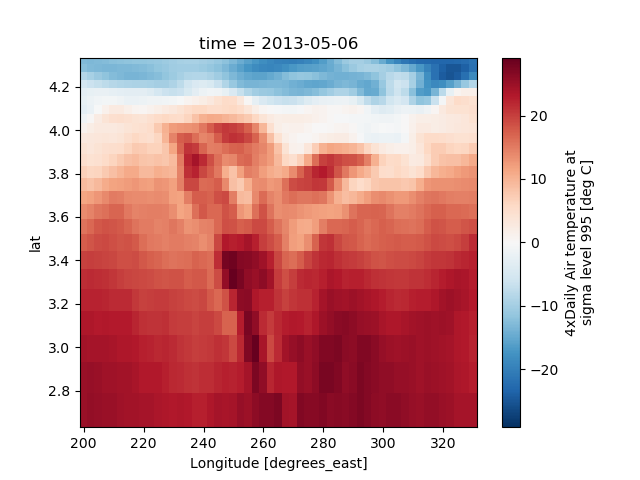### Calling Matplotlib¶

Since this is a thin wrapper around matplotlib, all the functionality of matplotlib is available.

```In : air2d.plot(cmap=plt.cm.Blues)

In : plt.title('These colors prove North America\nhas fallen in the ocean')
Out: Text(0.5, 1.0, 'These colors prove North America\nhas fallen in the ocean')

In : plt.ylabel('latitude')
Out: Text(0, 0.5, 'latitude')

In : plt.xlabel('longitude')
Out: Text(0.5, 0, 'longitude')

In : plt.tight_layout()

In : plt.draw()
```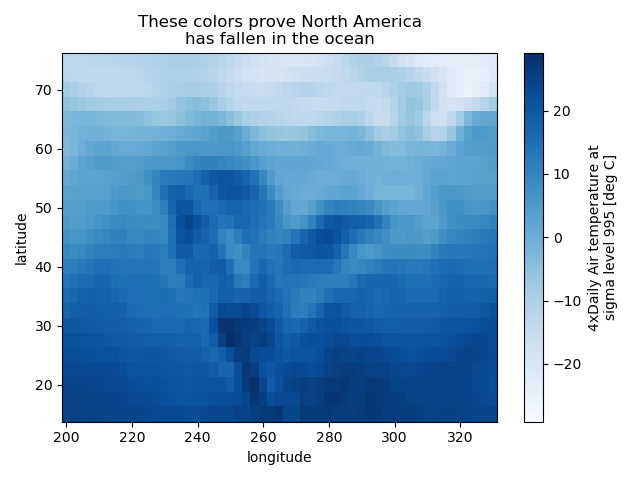Note

xarray methods update label information and generally play around with the axes. So any kind of updates to the plot should be done after the call to the xarray’s plot. In the example below, `plt.xlabel` effectively does nothing, since `d_ylog.plot()` updates the xlabel.

```In : plt.xlabel('Never gonna see this.')
Out: Text(0.5, 0, 'Never gonna see this.')

In : air2d.plot()

In : plt.draw()
```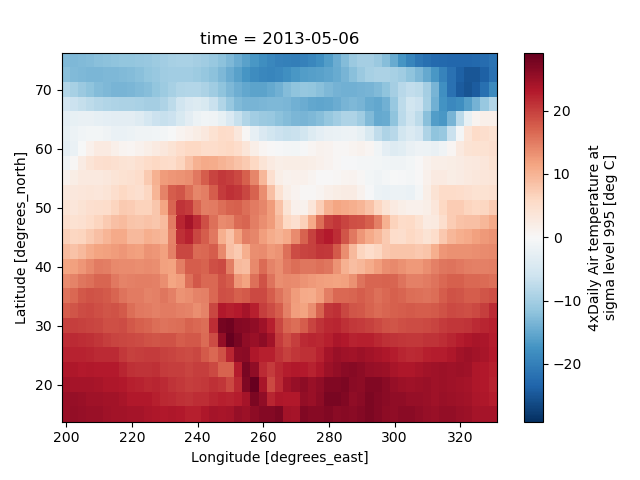### Colormaps¶

xarray borrows logic from Seaborn to infer what kind of color map to use. For example, consider the original data in Kelvins rather than Celsius:

```In : airtemps.air.isel(time=0).plot()
```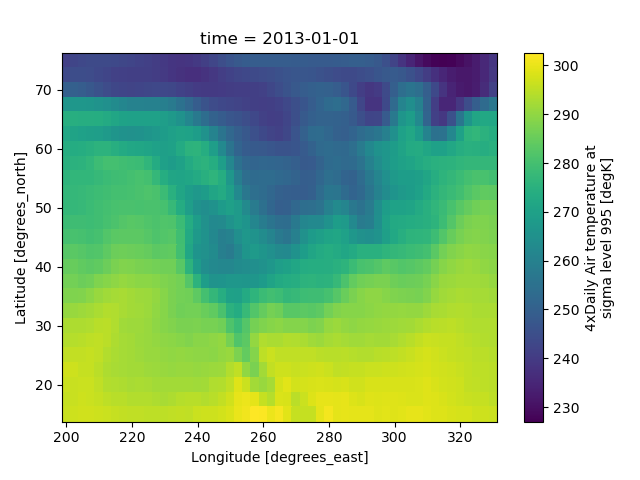The Celsius data contain 0, so a diverging color map was used. The Kelvins do not have 0, so the default color map was used.

### Robust¶

Outliers often have an extreme effect on the output of the plot. Here we add two bad data points. This affects the color scale, washing out the plot.

```In : air_outliers = airtemps.air.isel(time=0).copy()

In : air_outliers[0, 0] = 100

In : air_outliers[-1, -1] = 400

In : air_outliers.plot()
```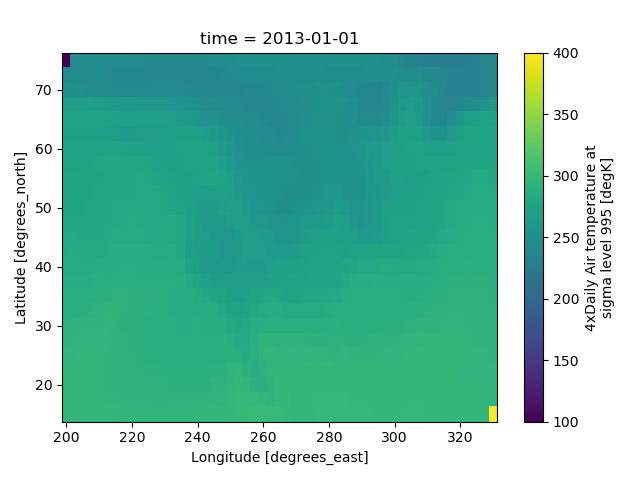This plot shows that we have outliers. The easy way to visualize the data without the outliers is to pass the parameter `robust=True`. This will use the 2nd and 98th percentiles of the data to compute the color limits.

```In : air_outliers.plot(robust=True)
```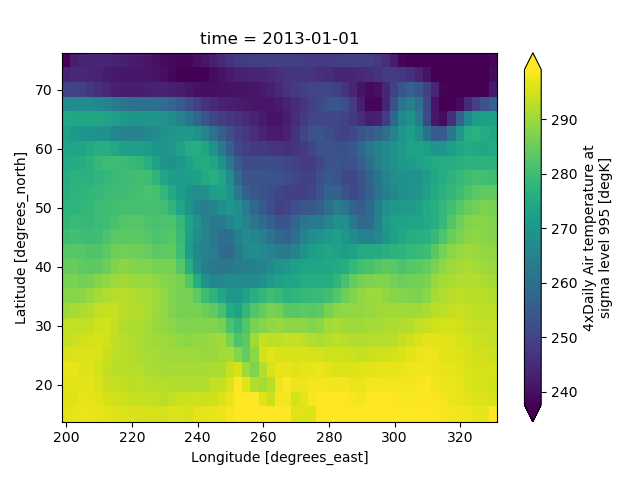Observe that the ranges of the color bar have changed. The arrows on the color bar indicate that the colors include data points outside the bounds.

### Discrete Colormaps¶

It is often useful, when visualizing 2d data, to use a discrete colormap, rather than the default continuous colormaps that matplotlib uses. The `levels` keyword argument can be used to generate plots with discrete colormaps. For example, to make a plot with 8 discrete color intervals:

```In : air2d.plot(levels=8)
```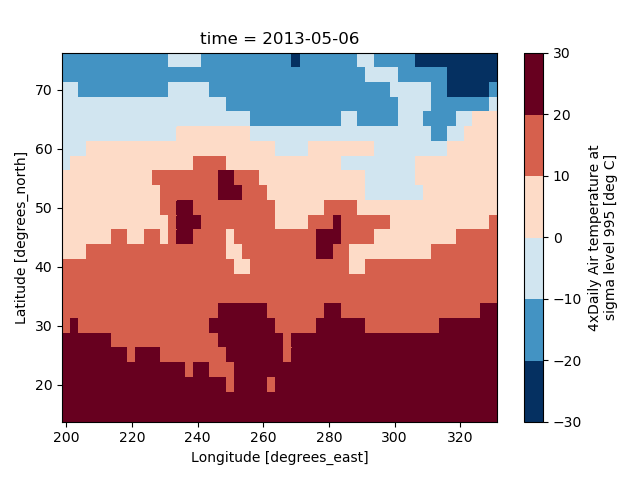It is also possible to use a list of levels to specify the boundaries of the discrete colormap:

```In : air2d.plot(levels=[0, 12, 18, 30])
```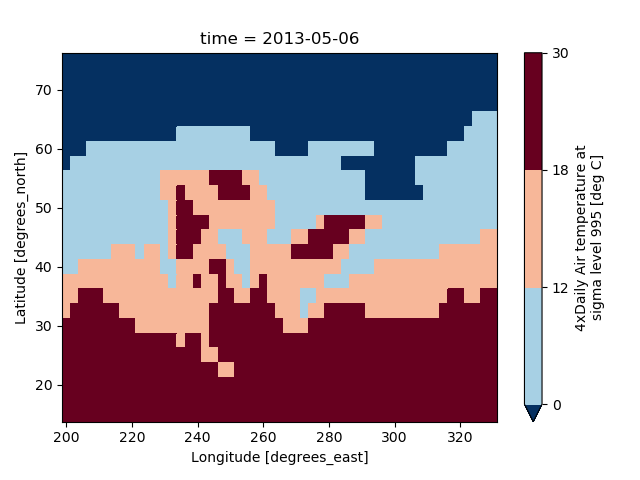You can also specify a list of discrete colors through the `colors` argument:

```In : flatui = ["#9b59b6", "#3498db", "#95a5a6", "#e74c3c", "#34495e", "#2ecc71"]

In : air2d.plot(levels=[0, 12, 18, 30], colors=flatui)
```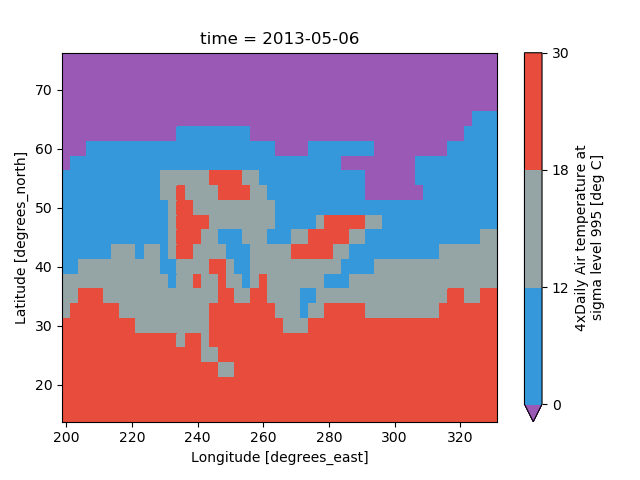Finally, if you have Seaborn installed, you can also specify a seaborn color palette to the `cmap` argument. Note that `levels` must be specified with seaborn color palettes if using `imshow` or `pcolormesh` (but not with `contour` or `contourf`, since levels are chosen automatically).

```In : air2d.plot(levels=10, cmap='husl')

In : plt.draw()
```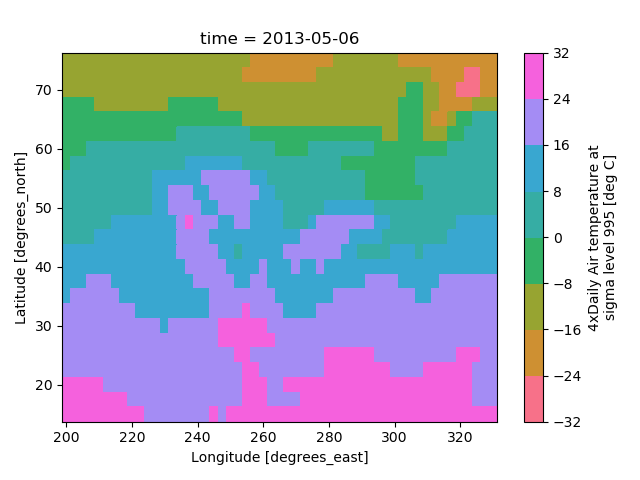## Faceting¶

Faceting here refers to splitting an array along one or two dimensions and plotting each group. xarray’s basic plotting is useful for plotting two dimensional arrays. What about three or four dimensional arrays? That’s where facets become helpful.

Consider the temperature data set. There are 4 observations per day for two years which makes for 2920 values along the time dimension. One way to visualize this data is to make a separate plot for each time period.

The faceted dimension should not have too many values; faceting on the time dimension will produce 2920 plots. That’s too much to be helpful. To handle this situation try performing an operation that reduces the size of the data in some way. For example, we could compute the average air temperature for each month and reduce the size of this dimension from 2920 -> 12. A simpler way is to just take a slice on that dimension. So let’s use a slice to pick 6 times throughout the first year.

```In : t = air.isel(time=slice(0, 365 * 4, 250))

In : t.coords
Out:
Coordinates:
* lat      (lat) float32 75.0 72.5 70.0 67.5 65.0 ... 25.0 22.5 20.0 17.5 15.0
* lon      (lon) float32 200.0 202.5 205.0 207.5 ... 322.5 325.0 327.5 330.0
* time     (time) datetime64[ns] 2013-01-01 ... 2013-11-09T12:00:00
```

### Simple Example¶

The easiest way to create faceted plots is to pass in `row` or `col` arguments to the xarray plotting methods/functions. This returns a `xarray.plot.FacetGrid` object.

```In : g_simple = t.plot(x='lon', y='lat', col='time', col_wrap=3)
```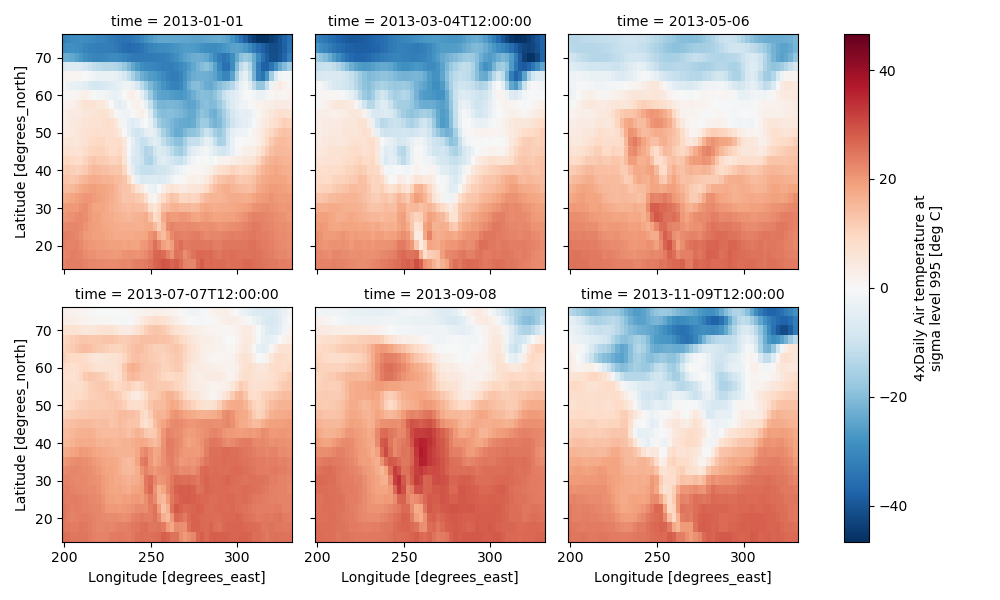Faceting also works for line plots.

```In : g_simple_line = t.isel(lat=slice(0,None,4)).plot(x='lon', hue='lat', col='time', col_wrap=3)
```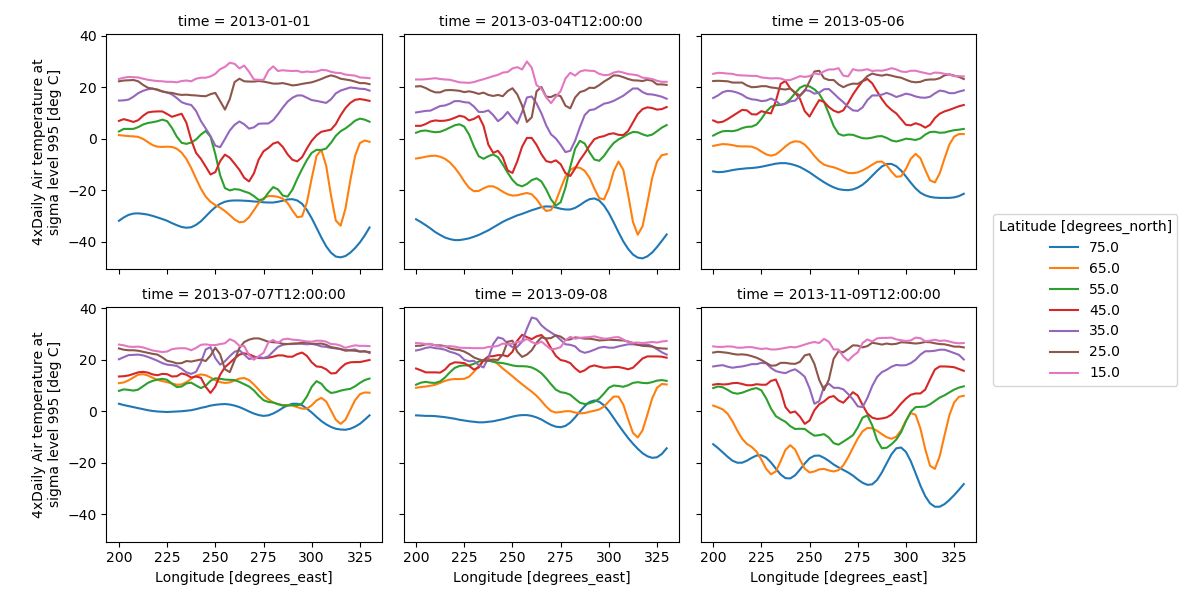### 4 dimensional¶

For 4 dimensional arrays we can use the rows and columns of the grids. Here we create a 4 dimensional array by taking the original data and adding a fixed amount. Now we can see how the temperature maps would compare if one were much hotter.

```In : t2 = t.isel(time=slice(0, 2))

In : t4d = xr.concat([t2, t2 + 40], pd.Index(['normal', 'hot'], name='fourth_dim'))

# This is a 4d array
In : t4d.coords
Out:
Coordinates:
* lat         (lat) float32 75.0 72.5 70.0 67.5 65.0 ... 22.5 20.0 17.5 15.0
* lon         (lon) float32 200.0 202.5 205.0 207.5 ... 325.0 327.5 330.0
* time        (time) datetime64[ns] 2013-01-01 2013-03-04T12:00:00
* fourth_dim  (fourth_dim) object 'normal' 'hot'

In : t4d.plot(x='lon', y='lat', col='time', row='fourth_dim')
Out: <xarray.plot.facetgrid.FacetGrid at 0x7f5b9318b860>
```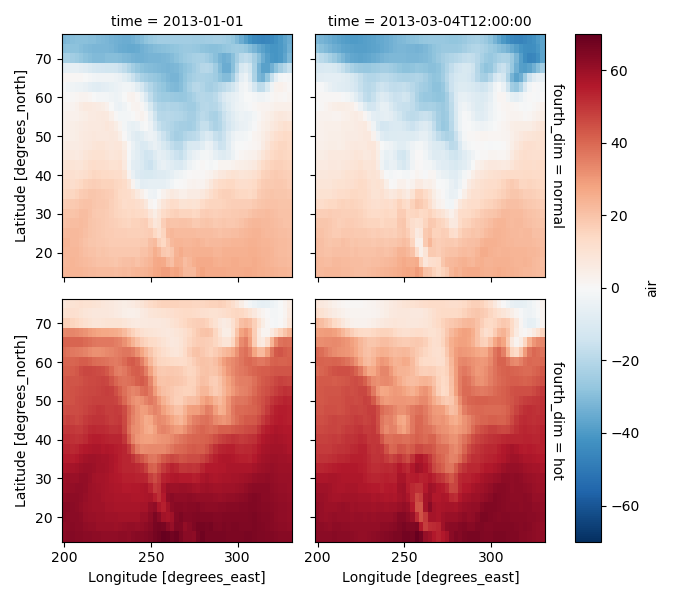### Other features¶

Faceted plotting supports other arguments common to xarray 2d plots.

```In : hasoutliers = t.isel(time=slice(0, 5)).copy()

In : hasoutliers[0, 0, 0] = -100

In : hasoutliers[-1, -1, -1] = 400

In : g = hasoutliers.plot.pcolormesh('lon', 'lat', col='time', col_wrap=3,
....:                                 robust=True, cmap='viridis',
....:                                 cbar_kwargs={'label': 'this has outliers'})
....:
```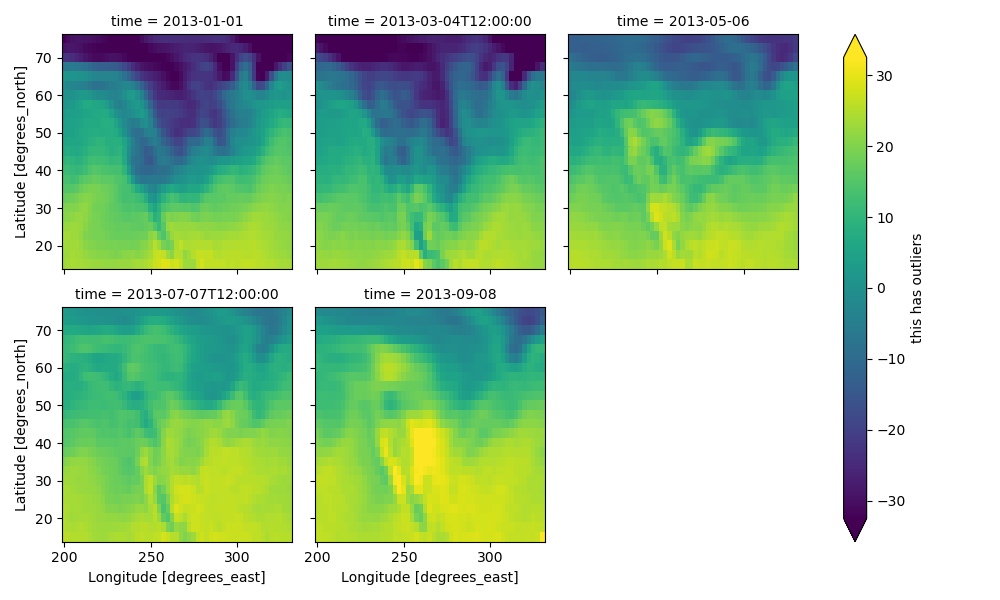### FacetGrid Objects¶

`xarray.plot.FacetGrid` is used to control the behavior of the multiple plots. It borrows an API and code from Seaborn’s FacetGrid. The structure is contained within the `axes` and `name_dicts` attributes, both 2d Numpy object arrays.

```In : g.axes
Out:
array([[<matplotlib.axes._subplots.AxesSubplot object at 0x7f5b92f91438>,
<matplotlib.axes._subplots.AxesSubplot object at 0x7f5b92fb3630>,
<matplotlib.axes._subplots.AxesSubplot object at 0x7f5b92f5b5c0>],
[<matplotlib.axes._subplots.AxesSubplot object at 0x7f5b92f88550>,
<matplotlib.axes._subplots.AxesSubplot object at 0x7f5b92f304e0>,
<matplotlib.axes._subplots.AxesSubplot object at 0x7f5b92ed9470>]], dtype=object)

In : g.name_dicts
Out:
array([[{'time': numpy.datetime64('2013-01-01T00:00:00.000000000')},
{'time': numpy.datetime64('2013-03-04T12:00:00.000000000')},
{'time': numpy.datetime64('2013-05-06T00:00:00.000000000')}],
[{'time': numpy.datetime64('2013-07-07T12:00:00.000000000')},
{'time': numpy.datetime64('2013-09-08T00:00:00.000000000')}, None]], dtype=object)
```

It’s possible to select the `xarray.DataArray` or `xarray.Dataset` corresponding to the FacetGrid through the `name_dicts`.

```In : g.data.loc[g.name_dicts[0, 0]]
Out:
<xarray.DataArray 'air' (lat: 25, lon: 53)>
array([[-100.      ,  -30.649994,  -29.649994, ...,  -40.350006,  -37.649994,
-34.550003],
[ -29.350006,  -28.649994,  -28.449997, ...,  -40.350006,  -37.850006,
-33.850006],
[ -23.149994,  -23.350006,  -24.259995, ...,  -39.949997,  -36.759995,
-31.449997],
...,
[  23.450012,   23.049988,   23.25    , ...,   22.25    ,   21.950012,
21.549988],
[  22.75    ,   23.049988,   23.640015, ...,   22.75    ,   22.75    ,
22.049988],
[  23.140015,   23.640015,   23.950012, ...,   23.75    ,   23.640015,
23.450012]], dtype=float32)
Coordinates:
* lat      (lat) float64 75.0 72.5 70.0 67.5 65.0 ... 25.0 22.5 20.0 17.5 15.0
* lon      (lon) float64 200.0 202.5 205.0 207.5 ... 322.5 325.0 327.5 330.0
time     datetime64[ns] 2013-01-01
Attributes:
long_name:     4xDaily Air temperature at sigma level 995
units:         deg C
precision:     2
GRIB_id:       11
GRIB_name:     TMP
var_desc:      Air temperature
dataset:       NMC Reanalysis
level_desc:    Surface
statistic:     Individual Obs
parent_stat:   Other
actual_range:  [185.16 322.1 ]
```

Here is an example of using the lower level API and then modifying the axes after they have been plotted.

```In : g = t.plot.imshow('lon', 'lat', col='time', col_wrap=3, robust=True)

In : for i, ax in enumerate(g.axes.flat):
....:     ax.set_title('Air Temperature %d' % i)
....:

In : bottomright = g.axes[-1, -1]

In : bottomright.annotate('bottom right', (240, 40))
Out: Text(240, 40, 'bottom right')

In : plt.draw()
```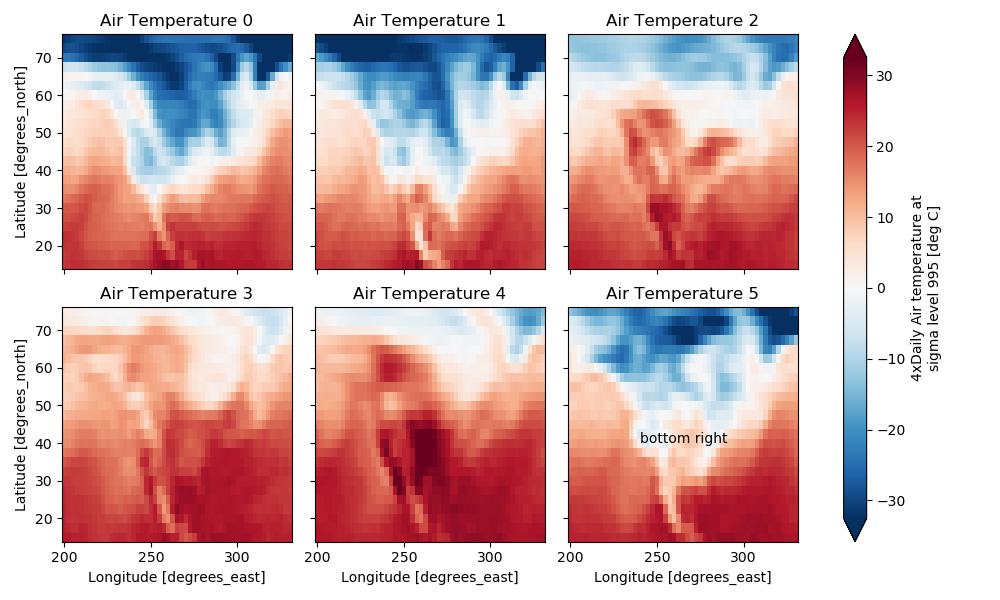TODO: add an example of using the `map` method to plot dataset variables (e.g., with `plt.quiver`).

## Maps¶

To follow this section you’ll need to have Cartopy installed and working.

This script will plot the air temperature on a map.

```In : import cartopy.crs as ccrs

In : air = xr.tutorial.open_dataset('air_temperature').air

In : ax = plt.axes(projection=ccrs.Orthographic(-80, 35))

In : air.isel(time=0).plot.contourf(ax=ax, transform=ccrs.PlateCarree());

In : ax.set_global(); ax.coastlines();
```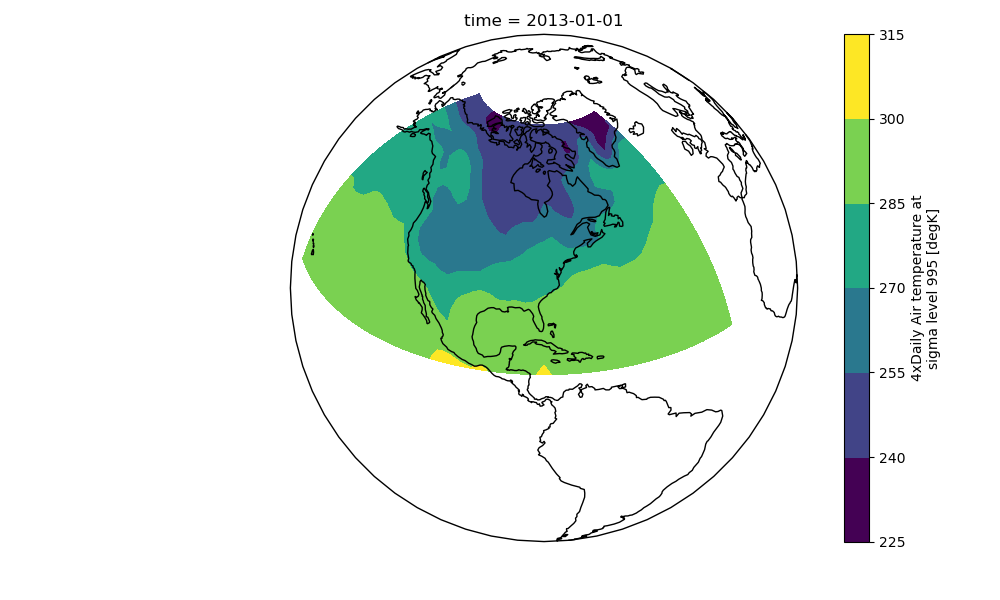When faceting on maps, the projection can be transferred to the `plot` function using the `subplot_kws` keyword. The axes for the subplots created by faceting are accessible in the object returned by `plot`:

```In : p = air.isel(time=[0, 4]).plot(transform=ccrs.PlateCarree(), col='time',
....:                                subplot_kws={'projection': ccrs.Orthographic(-80, 35)})
....:

In : for ax in p.axes.flat:
....:     ax.coastlines()
....:     ax.gridlines()
....:

In : plt.draw();
```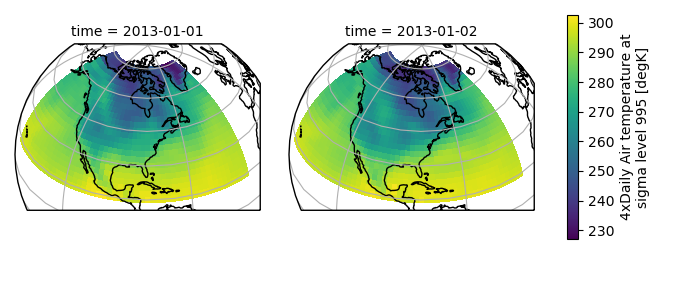## Details¶

### Ways to Use¶

There are three ways to use the xarray plotting functionality:

1. Use `plot` as a convenience method for a DataArray.

2. Access a specific plotting method from the `plot` attribute of a DataArray.

3. Directly from the xarray plot submodule.

These are provided for user convenience; they all call the same code.

```In : import xarray.plot as xplt

In : da = xr.DataArray(range(5))

In : fig, axes = plt.subplots(ncols=2, nrows=2)

In : da.plot(ax=axes[0, 0])
Out: [<matplotlib.lines.Line2D at 0x7f5b92a307f0>]

In : da.plot.line(ax=axes[0, 1])
Out: [<matplotlib.lines.Line2D at 0x7f5b92a306d8>]

In : xplt.plot(da, ax=axes[1, 0])
Out: [<matplotlib.lines.Line2D at 0x7f5b92a305f8>]

In : xplt.line(da, ax=axes[1, 1])
Out: [<matplotlib.lines.Line2D at 0x7f5b92a71f28>]

In : plt.tight_layout()

In : plt.draw()
```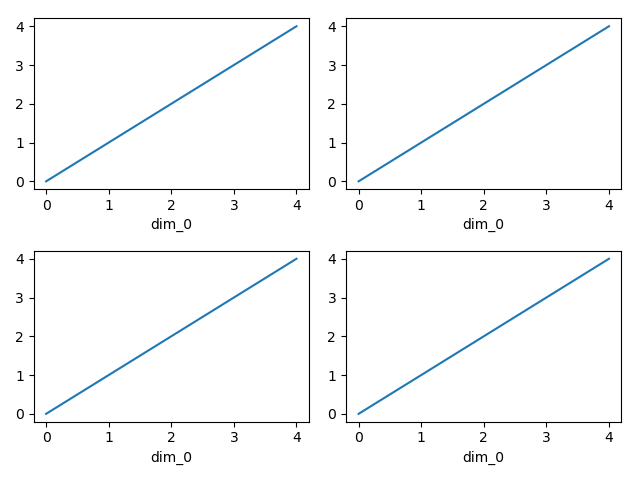Here the output is the same. Since the data is 1 dimensional the line plot was used.

The convenience method `xarray.DataArray.plot()` dispatches to an appropriate plotting function based on the dimensions of the `DataArray` and whether the coordinates are sorted and uniformly spaced. This table describes what gets plotted:

 Dimensions Plotting function 1 `xarray.plot.line()` 2 `xarray.plot.pcolormesh()` Anything else `xarray.plot.hist()`

### Coordinates¶

If you’d like to find out what’s really going on in the coordinate system, read on.

```In : a0 = xr.DataArray(np.zeros((4, 3, 2)), dims=('y', 'x', 'z'),
.....:                   name='temperature')
.....:

In : a0[0, 0, 0] = 1

In : a = a0.isel(z=0)

In : a
Out:
<xarray.DataArray 'temperature' (y: 4, x: 3)>
array([[1., 0., 0.],
[0., 0., 0.],
[0., 0., 0.],
[0., 0., 0.]])
Dimensions without coordinates: y, x
```

The plot will produce an image corresponding to the values of the array. Hence the top left pixel will be a different color than the others. Before reading on, you may want to look at the coordinates and think carefully about what the limits, labels, and orientation for each of the axes should be.

```In : a.plot()
```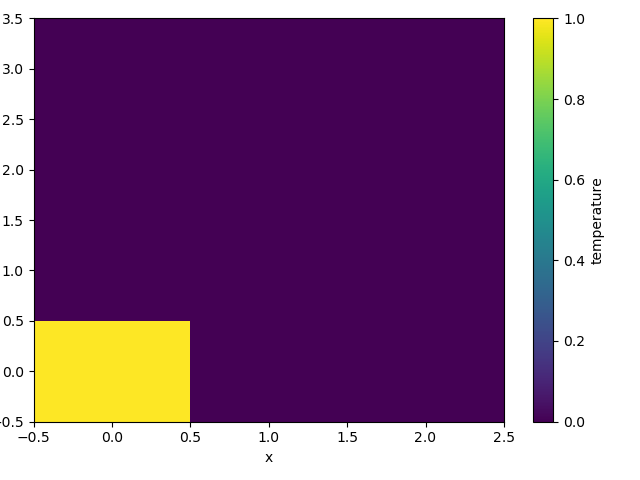It may seem strange that the values on the y axis are decreasing with -0.5 on the top. This is because the pixels are centered over their coordinates, and the axis labels and ranges correspond to the values of the coordinates.

### Multidimensional coordinates¶

You can plot irregular grids defined by multidimensional coordinates with xarray, but you’ll have to tell the plot function to use these coordinates instead of the default ones:

```In : lon, lat = np.meshgrid(np.linspace(-20, 20, 5), np.linspace(0, 30, 4))

In : lon += lat/10

In : lat += lon/10

In : da = xr.DataArray(np.arange(20).reshape(4, 5), dims=['y', 'x'],
.....:                   coords = {'lat': (('y', 'x'), lat),
.....:                             'lon': (('y', 'x'), lon)})
.....:

In : da.plot.pcolormesh('lon', 'lat');
```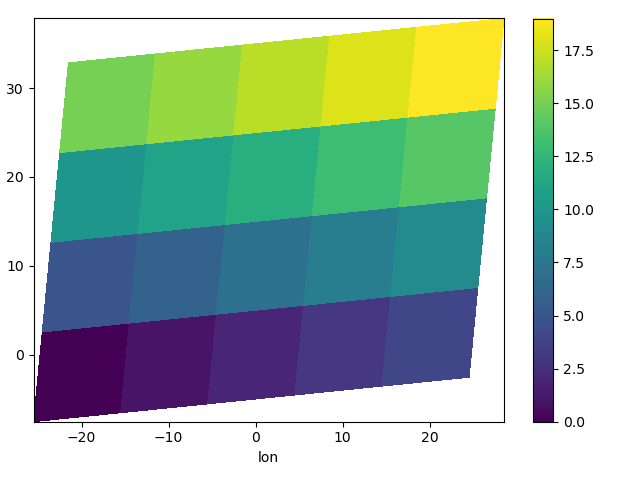Note that in this case, xarray still follows the pixel centered convention. This might be undesirable in some cases, for example when your data is defined on a polar projection (GH781). This is why the default is to not follow this convention when plotting on a map:

```In : import cartopy.crs as ccrs

In : ax = plt.subplot(projection=ccrs.PlateCarree());

In : da.plot.pcolormesh('lon', 'lat', ax=ax);

In : ax.scatter(lon, lat, transform=ccrs.PlateCarree());

In : ax.coastlines(); ax.gridlines(draw_labels=True);
```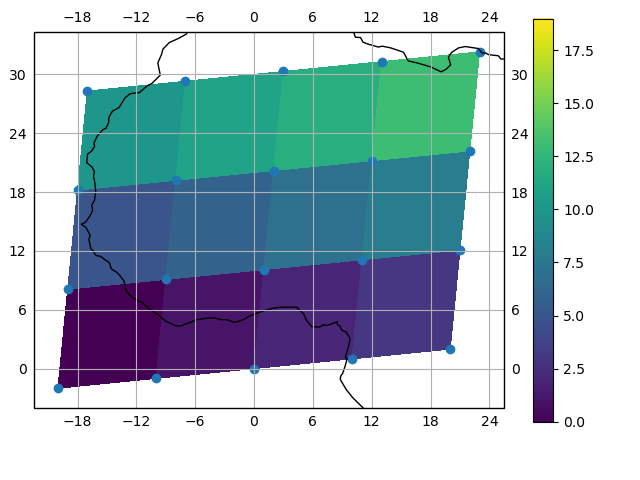You can however decide to infer the cell boundaries and use the `infer_intervals` keyword:

```In : ax = plt.subplot(projection=ccrs.PlateCarree());

In : da.plot.pcolormesh('lon', 'lat', ax=ax, infer_intervals=True);

In : ax.scatter(lon, lat, transform=ccrs.PlateCarree());

In : ax.coastlines(); ax.gridlines(draw_labels=True);
```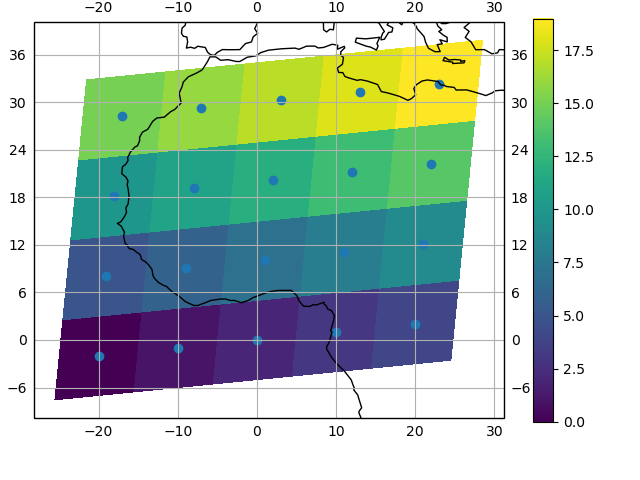Note

The data model of xarray does not support datasets with cell boundaries yet. If you want to use these coordinates, you’ll have to make the plots outside the xarray framework.

One can also make line plots with multidimensional coordinates. In this case, `hue` must be a dimension name, not a coordinate name.

```In : f, ax = plt.subplots(2, 1)

In : da.plot.line(x='lon', hue='y', ax=ax);

In : da.plot.line(x='lon', hue='x', ax=ax);
```